# NCERT SOLUTIONS FOR CLASS 11 CHEMISTRY CHAPTER 8-REDOX REACTIONS￼

Welcome to Swastik Classes! We are delighted to present to you the comprehensive NCERT Solutions for Class 11 Chemistry Chapter 8 – Redox Reactions. In this chapter, we embark on a fascinating journey into the world of oxidation-reduction reactions and their significance in chemistry.

Our meticulously crafted NCERT Solutions provide a step-by-step approach to understanding the fundamental concepts and principles of redox reactions. Whether you’re a student seeking clarity or an educator looking for reliable teaching resources, our solutions offer a comprehensive and easy-to-follow guide.

Through our meticulously curated explanations, examples, and practice questions, we aim to help you build a solid foundation in redox reactions. We delve into topics such as oxidation and reduction, oxidation number, balancing redox reactions by the ion-electron method, and much more.

Our team of experienced educators and subject matter experts has crafted these solutions with a student-centric approach, ensuring that you not only grasp the theoretical aspects but also develop problem-solving skills. By engaging with our solutions, you’ll gain the confidence to tackle a wide range of questions and excel in your examinations.

At Swastik Classes, we believe in making learning enjoyable and accessible. Our NCERT Solutions are designed to simplify complex concepts, foster critical thinking, and stimulate your curiosity about the fascinating world of chemistry. We provide you with the necessary tools to excel academically and develop a lifelong love for the subject.

Embrace the enriching experience of learning redox reactions with our NCERT Solutions for Class 11 Chemistry Chapter 8. Let Swastik Classes be your trusted companion on your educational journey. Get ready to unlock the mysteries of redox reactions and achieve academic excellence!

## NCERT SOLUTIONS FOR CLASS 11 CHEMISTRY CHAPTER 8-REDOX REACTIONS – Exercises

### Chapter-8 Reodx Reactions

1. Assign oxidation number to the underlined elements in each of the following species:
(a)NaH2 P O4
(b)NaH SO4
(c)H4 P2O7
(d)K2MnO4
(e)CaO2
(f)NaBH4
(g)H2S2O7
(h)KAl(SO4)⋅ 12H2O

(a) Let the oxidation number of P be x.
We know that,
Oxidation number of Na = +1
Oxidation number of H = +1
Oxidation number of O = –2
NaH2PO4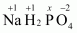Then, we have
1(+1)+ 2 (+1) + 1(x) + 4(−2)=0
⇒1+2+x−8=0
⇒x=+5
Hence, the

(b)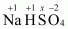Then, we have
1(+1) +1(+1) + 1(x) +4(−2)=0
⇒1+1 + x− 8 = 0

⇒x=+6
Hence, the oxidation number of S is + 6.

(c) H4P2O7

Then, we have
4(+1) + 2(x) + 7(−2)=0
⇒4+2x−14=0
⇒2x= +10
⇒x=+5
Hence, the oxidation number of P is + 5.

(d) K2MnO4

Then, we have
2(+1) + x+ 4(−2)=0
⇒2+x−8=0
⇒x=+6
Hence, the oxidation number of Mn is + 6.

(e)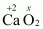Then, we have
(+2) +2(x)=0
⇒2+2x=0
⇒x=−1

(f) 9NaBH4
Then, we have
1(+1) +1(x)+4(−1)=0
⇒1+x−4=0
⇒x=+3
Hence, the oxidation number of B is + 3.

(g) H2S2O7
Then, we have
2(+1) +2(x) +7(−2)=0
⇒2 +2x− 14=0
⇒2x=12
⇒x=+6
Hence, the oxidation number of S is + 6.

(h ) KAl (SO4)2 ⋅ 12H2O
Then, we have
1(+1) 1(+3)+ 2(x) +8(−2) +24(+1) +12(−2) =0
⇒1 +3 +2x −16 +24 −24=0
⇒2x=12
⇒x=+6
Or,
We can ignore the water molecule as it is a neutral molecule. Then, the sum of the oxidation numbers of all atoms of the water molecule may be taken as zero. Therefore, after ignoring the water molecule, we have
1(+1) +1(+3) +2(x) +8(−2) =0
⇒1+3+2x−16=0
⇒2x=12
⇒x=+6
Hence, the oxidation number of S is + 6.
oxidation number of P is +5.

2. What are the oxidation numbers of the underlined elements in each of the following and how do you rationalise your results?
(a) Kl3
(b) H2S4O6
(c)Fe3O4
(d)CH3 CHOH
(e)CH3 COOH

(a) Kl3
In  Kl3,the oxidation number (O.N.) of K is +1. Hence, the average oxidation number of I is −1/3 .However, O.N. cannot be fractional. Therefore, we will have to consider the structure of KI3to find the oxidation states.
In a Kl3 molecule, an atom of iodine forms a coordinate covalent bond with an iodine molecule. Hence, in a  Kl3 molecule, the O.N. of the two I atoms forming the l2> molecule is 0, whereas the O.N. of the I atom forming the coordinate bond is as 1.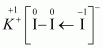(b)H2S4O6However, O.N. cannot be fractional. Hence, S must be present in different oxidation states in the molecule.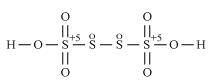The O.N. of two of the four S atoms is +5 and the O.N. of the other two S atoms is 0.

(c)Fe3O4
On taking the O.N. of O as 2, the O.N. of Fe is found to be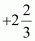.However, O.N. cannot be fractional.
Here, one of the three Fe atoms exhibits the O.N. of +2 and the other two Fe atoms exhibit the O.N. of +3.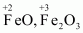(d) CH3 CH2 OH
2(x) +6(+1) +1(−2)=0
2x+4=0
x=−2
Hence, the O.N. of C is −2

(e) CH3 COOH
2(x) +4(+1) +2(−2)=0
2x=0
x=0
However, 0 is average O.N. of C.
The two carbon atoms present in this molecule are present in different environments. Hence, they cannot have the same oxidation number. Thus, C exhibits the oxidation states of +2 and −2 in CH3 COOH.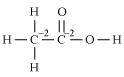3. Justify that the following reactions are redox reactions:
(a) CuO(s) + H2(g) → Cu(s) + H2O(g)
(b) Fe2O3(s) + 3cO(g) → 2Fe(s) + 3CO2(g)
(c) 4BCl3(g) + 3LiAlH4(s)→ 2B2H6(g) + 3LiCl(s)+3AlCl3(s)
(d) 2K(s) + F2(g) → 2K + F−(s)
(e) 4NH3(g) + 5O2(g) → 4NO(g)+6H2O(g)

(a) CuO(s)+H2(g) longrightarrow Cu(s)+H2O(g)
Let us write the oxidation number of each element involved in the given reaction as: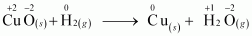Here, the oxidation number of Cu decreases from +2 in CuO to 0 in Cu i.e., CuO is reduced to Cu. Also, the oxidation number of H increases from 0 in H2 to +1 in H2O i.e., H2 is oxidized to H2O .Hence, this reaction is a redox reaction.

(b) Fe2O3(s) + 3CO(g)⟶ 2Fe(s) + 3CO2(g)
Let us write the oxidation number of each element in the given reaction as: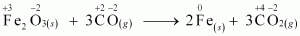Here, the oxidation number of Fe decreases from +3 in Fe2O3 to 0 in Fe i.e.,  Fe2O3 is reduced to Fe. On the other hand, the oxidation number of C increases from +2 in CO to +4 in CO2 i.e., CO is oxidized to  CO2 .Hence, the given reaction is a redox reaction.

(c)  4BCl3(g) + 3LiAlH4(s) ⟶ 2B2H6(g) + 3LiCl(s) +  3AlCl3(s)
The oxidation number of each element in the given reaction can be represented as: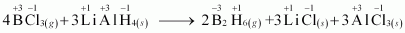In this reaction, the oxidation number of B decreases from +3 in BCl3 to –3 in B2H6 .i.e., BCl3 is reduced toB2H6 . Also, the oxidation number of H increases from –1 in LiAlH4 to +1 in B2H6 i.e., LiAlH4 is oxidized to B2H6 .Hence, the given reaction is a redox reaction.

(d) 2K(s) + F2(g) ⟶ 2K + F−
The oxidation number of each element in the given reaction can be represented as: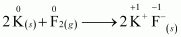(e) 4NH3(g) + 5O2(g) ⟶4NO(g) + 6H2O(g)
The oxidation number of each element in the given reaction can be represented as:
Here, the oxidation number of N increases from –3 in NH3 to +2 in NO. On the other hand, the oxidation number of O2 decreases from 0 in Oto –2 in NO and H2O i.e., O2 is reduced. Hence, the given reaction is a redox reaction.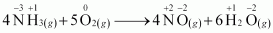4. Fluorine reacts with ice and results in the change:
H2O(s) + F2(g) → HF(g)+ HOF(g)
Justify that this reaction is a redox reaction:

Let us write the oxidation number of each atom involved in the given reaction above its symbol as: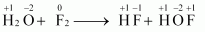Here, we have observed that the oxidation number of F increases from 0 in F2 to +1 in HOF. Also, the oxidation number decreases from 0 in F2 to –1 in HF. Thus, in the above reaction, F is both oxidized and reduced. Hence, the given reaction is a redox reaction.

5. Calculate the oxidation number of sulphur, chromium and nitrogen in H2SO5,Cr2O2 −7  and NO3. Suggest structure of these compounds. Count for the fallacy.

(i) H2SO5
2(+1) + 1(x) +5(−2)=0
⇒2 +x −10=0
⇒x=+8
However, the O.N. of S cannot be +8. S has six valence electrons. Therefore, the O.N. of S cannot be more than +6.
The structure of H2SO5 is shown as follows: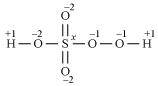Now, 2(+1) +1(x) +3(−2) +2(−1) =0
⇒2 +x−6 −2=0
⇒x=+6
Therefore, the O.N. of S is +6.

(ii)Cr2O2−7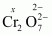2(x) +7(−2) =−2
⇒2x−14 =−2
⇒x=+6
Here, there is no fallacy about the O.N. of Cr inCr2O2−7
The structure of Cr2O2−7 is shown as follows: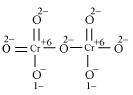Here, each of the two Cr atoms exhibits the O.N. of +6.

(iii) NO3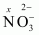1(x)+3(−2)=−1
⇒x−6=−1
⇒x=+5
Here, there is no fallacy about the O.N. of N in NO3
The structure of  NO3 is shown as follows: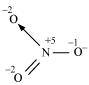The N atom exhibits the O.N. of +5.

6. Write the formula for the following compounds:
(a)Mercury(II) chloride
(b)Nickel(II) sulphate
(c)Tin(IV) oxide
(d)Thallium(I) sulphate
(e)Iron(III) sulphate
(f)Chromium(III) oxide

(a)Mercury (II) chloride: HgCl2
(b)Nickel (II) sulphate: NiSO4
(c)Tin (IV) oxide: SnO2
(d)Thallium (I) sulphate: TI2SO4
(e)Iron (III) sulphate: Fe2(SO4)3
(f)Chromium (III) oxide: Cr2O3

7. Suggest a list of the substances where carbon can exhibit oxidation states from –4 to +4 and nitrogen from –3 to +5.

The substances where carbon can exhibit oxidation states from –4 to +4 are listed in the following table.

The substances where nitrogen can exhibit oxidation states from –3 to +5 are listed in the following table.

The substances where nitrogen can exhibit oxidation states from –3 to +5 are listed in the following table.

8. While sulphur dioxide and hydrogen peroxide can act as oxidising as well as reducing agents in their reactions, ozone and nitric acid act only as oxidants. Why?

In sulphur dioxide (SO2), the oxidation number (O.N.) of S is +4 and the range of the O.N. that S can have is from +6 to –2.

Therefore, SO2 can act as an oxidising as well as a reducing agent.

In hydrogen peroxide (H2O2), the O.N. of O is –1 and the range of the O.N. that O can have is from 0 to –2. O can sometimes also attain the oxidation numbers +1 and +2. Hence, H2O2 can act as an oxidising as well as a reducing agent.

In ozone (O3), the O.N. of O is zero and the range of the O.N. that O can have is from 0 to –2. Therefore, the O.N. of O can only decrease in this case. Hence, Oacts only as an oxidant.

In nitric acid (HNO3), the O.N. of N is +5 and the range of the O.N. that N can have is from +5 to –3. Therefore, the O.N. of N can only decrease in this case. Hence, HNO3 acts only as an oxidant.

9. Consider the reactions:
(a) 6 CO2(g) + 6H2O(l) → C6 H12 O6(aq) + 6O2(g)
(b) O3(g) + H2O2(l) → H2O(l) + 2O2(g)
Why it is more appropriate to write these reactions as

(a) 6CO2(g) + 12H2O(l) → C6 H12 O6(aq) + 6H2O(l) + 6O2(g)
(b) O3(g) + H2O(l) → H2O(l) + O2(g) + O2(g)
Also suggest a technique to investigate the path of the above (a) and (b) redox reactions.

(a)The process of photosynthesis involves two steps.
Step 1:
H2O decomposes to give H2 and O2.
2H2O(l) ⟶ 2H2(s) + O2(s)

Step 2:
The H2 produced in step 1 reduces CO2, thereby producing glucose (C6H12O6) and H2O.
Now, the net reaction of the process is given as: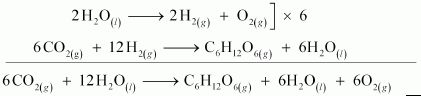It is more appropriate to write the reaction as given above because water molecules are also produced in the process of photosynthesis.
The path of this reaction can be investigated by using radioactive H2O18 in place of H2O.

(b) Ois produced from each of the two reactants Oand H2O2. For this reason, O2 is written twice.
The given reaction involves two steps. First, O3 decomposes to form O2 and O. In the second step, H2O2 reacts with the O produced in the first step, thereby producing H2O and O2.

The path of this reaction can be investigated by using H2O182 or O183.

10. The compound AgFis an unstable compound. However, if formed, the compound acts as a very strong oxidizing agent. Why?

Answer: The oxidation state of Ag in AgF2 is +2. But, +2 is an unstable oxidation state of Ag. Therefore, whenever AgF2 is formed, silver readily accepts an electron to form Ag+. This helps to bring the oxidation state of Ag down from +2 to a more stable state of +1. As a result, AgF2 acts as a very strong oxidizing agent.

11. Whenever a reaction between an oxidising agent and a reducing agent is carried out, a compound of lower oxidation state is formed if the reducing agent is in excess and a compound of higher oxidation state is formed if the oxidising agent is in excess. Justify this statement giving three illustrations.

Answer: Whenever a reaction between an oxidising agent and a reducing agent is carried out, a compound of lower oxidation state is formed if the reducing agent is in excess and a compound of higher oxidation state is formed if the oxidising agent is in excess. This can be illustrated as follows:

(i)P4 and F2 are reducing and oxidising agents respectively.
If an excess of P4 is treated with F2, then PF3 will be produced, wherein the oxidation number (O.N.) of P is +3.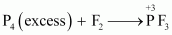However, if P4 is treated with an excess of F2, then PF5 will be produced, wherein the O.N. of P is +5.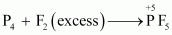(ii)K acts as a reducing agent, whereas O2 is an oxidising agent.
If an excess of K reacts with O2, then K2O will be formed, wherein the O.N. of O is –2.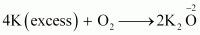However, if K reacts with an excess of O2, then K2O2 will be formed, wherein the O.N. of O is –1.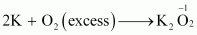(iii)C is a reducing agent, while O2 acts as an oxidising agent.
If an excess of C is burnt in the presence of insufficient amount of O2, then CO will be produced, wherein the O.N. of C is +2.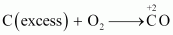On the other hand, if C is burnt in an excess of O2, then CO2 will be produced, wherein the O.N. of C is +4.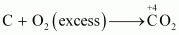12. How do you count for the following observations?
(a) Though alkaline potassium permanganate and acidic potassium permanganate both are used as oxidants, yet in the manufacture of benzoic acid from toluene we use alcoholic potassium permanganate as an oxidant. Why? Write a balanced redox equation for the reaction.
(b) When concentrated sulphuric acid is added to an inorganic mixture containing chloride, we get colourless pungent smelling gas HCl, but if the mixture contains bromide then we get red vapour of bromine. Why?

(a) In the manufacture of benzoic acid from toluene, alcoholic potassium permanganate is used as an oxidant because of the following reasons.
(i) In a neutral medium, OH– ions are produced in the reaction itself. As a result, the cost of adding an acid or a base can be reduced.
(ii) KMnO4 and alcohol are homogeneous to each other since both are polar. Toluene and alcohol are also homogeneous to each other because both are organic compounds. Reactions can proceed at a faster rate in a homogeneous medium than in a heterogeneous medium. Hence, in alcohol, KMnO4 and toluene can react at a faster rate.
The balanced redox equation for the reaction in a neutral medium is give as below:

(b) When conc.H2SO4 is added to an inorganic mixture containing bromide, initially HBr is produced. HBr, being a strong reducing agent reduces H2SO4 to SO2 with the evolution of red vapour of bromine.
2NaBr + 2H2SO4 ⟶2NaHSO4+2HBr
2HBr + H2SO4 ⟶ Br+ SO2 + 2H2O
But, when conc. H2SO4 is added to an inorganic mixture containing chloride, a pungent smelling gas (HCl) is evolved. HCl, being a weak reducing agent, cannot reduce  H2SO4 to SO2.
2NaCl+2H2SO4⟶2NaHSO4+2HCl.

13. Identify the substance oxidised, reduced, oxidising agent and reducing agent for each of the following reactions:
(a)2AgBr(s) + C6H6O2(aq) → 2Ag(s) + 2HBr(aq) + C6H4O2(aq)
(b)HCHO(l) + 2[ Ag (NH3)] + (aq) + 3OH − (aq)→ 2Ag(s) + HCOO − (aq) + 4NH3(aq) + 2H2O(l)
(c)HCHO (1) + 2Cu2 +(aq) + 5OH − (aq)→ Cu2O(s) + HCOO − (aq)+3H2O(l)
(d) N2H4(l) + 2H2O2(l) → N2(g) + 4H2O(l)
(e) Pb(s) + PbO2(s) + 2H2SO4 (aq) → 2PbSO4(s) + 2H2O(l)

(a) Oxidised substance →C6H6O2
Reduced substance → AgBr
Oxidising agent → AgBr
Reducing agent → C6H6O2

(b)Oxidised substance → HCHO
Reduced substance →[Ag (NH)2]+
Oxidising agent → [Ag (NH3)2]+
Reducing agent → HCHO

(c) Oxidised substance → HCHO
Reduced substance → Cu2+
Oxidising agent → Cu2+
Reducing agent → HCHO

(d) Oxidised substance →N2H4
Reduced substance → H2O2
Oxidising agent → H2O2
Reducing agent → N2H4

(e) Oxidised substance → Pb
Reduced substance → PbO2
Oxidising agent → PbO2
Reducing agent → Pb

14. Consider the reactions:
2S2O− 3(aq) + I2(s) → S4O2 − 6(aq)+2I − (aq)
S2O2 − 3aq) + 2Br2(l)+5H2O(l) → 2SO2 − 4(aq) + 4Br − (aq) + 10H+

Ans : The average oxidation number (O.N.) of S in S2O2−3 is +2. Being a stronger oxidising agent than I2, Br2 oxidises S2O2−3 to SO2−4 , in which the O.N. of S is +6. However, I2 is a weak oxidising agent. Therefore, it oxidises S2O2−3 to
S4O2−, in which the average O.N. of S is only +2.5. As a result, S2O2−3 reacts differently with iodine and bromine.

15. Justify giving reactions that among halogens, fluorine is the best oxidant and among hydrohalic compounds, hydroiodic acid is the best reductant.

Fcan oxidize Cl− to Cl2,Br− to Br2, and I− to I2
On the other hand, Cl2,Br2, and I2 cannot oxidize F− to F2 .The oxidizing power of halogens increases in the order of I< Br2 < Cl2 < F. Hence, fluorine is the best oxidant among halogens.
HI and HBr can reduce H2SO4 to SO2, but HCl and HF cannot. Therefore, HI and HBr are stronger reductants than HCl and HF.
2HI + H2SO4 ⟶ I2 + SO+ 2H2O
2HBr + H2SO⟶ Br2 + SO2+2H2O
1 can reduce Cu2+ to Cu+, but Br
4I− (aq) + 2Cu2 + (aq) ⟶ Cu2I2(s) + I2(aq)

Hence, hydroiodic acid is the best reductant among hydrohalic compounds.
Thus, the reducing power of hydrohalic acids increases in the order of
HF < HCl < HBr < HI.

16. Why does the following reaction occur?
XeO4− 6(aq) +2F − (aq) + 6H+ (aq) → XeO3(g) + F2(g) + 3H2O(l)
What conclusion about the compound Na4XeO6 (of which XeO4−6 is a part) can be drawn from the reaction.

The given reaction occurs because XeO4−6 oxidises F− and F− reduces XeO4−6.
XeO + 6(aq)+ 2F − 1(aq) + 6H + (aq)-> X3(g)+0F2(x)+3H2O(l)
In this reaction, the oxidation number (O.N.) of Xe decreases from +8 in
XeO4−6 to +6 in XeO3 and the O.N. of F increases from –1 in F to O in F2
Hence, we can conclude that Na4 XeO6 is a stronger oxidising agent than F.

17. consider the reactions:
(a) H3PO2 (aq) + 4AgNO3 (aq) + 2H2O(l) → H3PO4 (aq) + 4Ag(s)+ 4HNO3 (aq)
(b) H3PO2 (aq) + 2CuSO4 (aq) + 2H2O(I) → H3PO4(aq) + 2Cu(s) + H2SO4(aq)
(c) C6H5 CHO(I) + 2[Ag (NH3)2] + (aq)+3OH − (aq) → C6H5COO − (aq) + 2Ag(s) +4NH3(aq)+2H2O(l)
(d) C6H5CHO(l) + 2Cu+ (aq) +5OH  (aq) → No change  observed.

Cu2+ and Cu2+ act as oxidising agents in reactions

(a) and

(b)respectively. In reaction

(c), Cu2+ oxidises C6H5 CHO to C6H5 COO, but in reaction

(d), Cu2+ cannot oxidise C6H5CHO.
Hence, we can say that  Cu2+ is a stronger oxidising agent than Cu2+.

18. Balance the following redox reactions by ion-electron method:
(a) MnO − 4(aq) + I(aq) → MnO2(s) + I2(s) ( in basic medium)
(b) MnO − 4(aq) + SO2(g) → Mn2+(aq) + HSO− 4(aq)
(c) H2O(aq) + Fe2+ (aq) → Fe3+ (aq) + H2O(l) (in acidic  solution)
Cr2O2−7 + SO2(g) → Cr3+(aq) + SO2−4 (aq) ( in acidic solution )

(a) Step 1:
The two half reactions involved in the given reaction are:

Oxidation half reaction: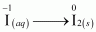Reduction half reaction: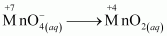Step 2: Balancing I in the oxidation half reaction, we have:
2I−(aq)⟶I2(s)

Now, to balance the charge, we add 2 e to the RHS of the reaction.
2I(aq)⟶I2(x)+2e

Step 3: In the reduction half reaction, the oxidation state of Mn has reduced from +7 to +4. Thus, 3 electrons are added to the LHS of the reaction.
MnO4(aq)+3e⟶MnO2(aq)

Now, to balance the charge, we add 4 OH ions to the RHS of the reaction as the reaction is taking place in a basic medium.
MnO4(oq) + 3e⟶MnO2(cq) + 4OH

Step 4: In this equation, there are 6 O atoms on the RHS and 4 O atoms on the LHS. Therefore, two water molecules are added to the LHS.
MnO4(cq) + 2H2O + 3e⟶ MnO2(ca) + 4OH

Step 5: Equalising the number of electrons by multiplying the oxidation half reaction by 3 and the reduction half reaction by 2, we have:
6I−(aq)⟶ 3I2(s) + 6e
2MnO4(aq) + 4H2O + 6e⟶ 2MnO2(s) + 8OH(aq)

Step 6: Adding the two half reactions, we have the net balanced redox reaction as:
6I(eq) + 2MnO − 4(cy) + 4H2O(l) ⟶  3I2(s) + 2MnO2(s) + 8OH(aq)

(b)Following the steps as in part (a), we have the oxidation half reaction as:
SO2(x)+2H2O(l)

And the reduction half reaction as:
MnO− 4(αq) + 8H + (aq) + 5e⟶ Mn2+(aq) + 4H2O(l)

Multiplying the oxidation half reaction by 5 and the reduction half reaction by 2, and then by adding them, we have the net balanced redox reaction as:
2MnO − 4(aq) + 5SO2(g) + 2H2O(l) +H+(aq) ⟶ 2Mn2+(aq) + 5HSO4(aq)

(c) Following the steps as in part (a), we have the oxidation half reaction as:
Fe2+(αq)⟶Fe3+(aq)+e−

And the reduction half reaction as:
H2O2(aq) + 2H+(aq) + 2e⟶ 2H2O(l)

Multiplying the oxidation half reaction by 2 and then adding it to the reduction half reaction, we have the net balanced redox reaction as:
H2O2(aq) + 2Fe2+(aq) + 2H+(aq) ⟶ 2Fe3+(aq) + 2H2O(l)

(d) Following the steps as in part (a), we have the oxidation half reaction as:
SO2(g)+2H2Ol) ⟶ SO2−4 + 4H+(aq)+2e

And the reduction half reaction as:
Cr2O2−7 + 14H+(aq) + 6e ⟶ 2Cr3+(aq)+7H2O(l)

Multiplying the oxidation half reaction by 3 and then adding it to the reduction half reaction, we have the net balanced redox reaction as:
Cr2O2−7 + 3SO2(g) + 2H+(aq) ⟶ 2Cr3+(aq) + 3SO2− + H2O(l)

19. Balance the following equations in basic medium by ion-electron method and oxidation number methods and identify the oxidising agent and the reducing agent.
P4(s) + OH−(oq)⟶ PH3(g) + HPO2
N2H4(l) + ClOaq ⟶ NO(g) + Cl(g)
Cl2O7(g) + H2O2(aq) ⟶ ClO2(aq) + O2(g)+ H+(aq)

(a)The O.N. (oxidation number) of P decreases from 0 in P4 to –3 in PH3 and increases from 0 inP4 to + 2 inHPO2. Hence, P4acts both as an oxidizing agent and a reducing agent in this reaction.
Ion–electron method :
The oxidation half equation is:
P4s → HPO2(aq)
The P atom is balanced as:
P4→ 4HPO2– (aq)
The O atom is balanced by adding 8 H2O molecules:
P+ 8H2O →  4(aq)
The H atom is balanced by adding 12 H+ ions:
P4 + 8H2O → 4HPO − 2(aq) + 12H+
The charge is balanced by adding e as:
P4 + 8H2O → 4HPO2 (aq) + 12H++ 8e−…(i)

The reduction half equation is :
P4(s) ⟶ PH3(g)
The P atom is balanced as:
P4→ 4PH3(g)
The H is balanced by adding 12 H+ as:
P4 + 12H+ → 4PH3(g)
The charge is balanced by adding 12e– as:
P4+ 12H++12e→ 4PH3(g) …(ii)
By multiplying equation (i) with 3 and (ii) with 2 and then adding them, the balanced chemical equation can be obtained as:
5P4(s) + 24H2O → 12HPO2– + 8PH3(g) + 12H+
As, the medium is basic, add 12OH– both sides as:
5P4(s) +12H2O + 12OH →12HPO2+8PH3(g)
This is the required balanced equation.
Oxidation number method:
Let, total no of P reduced = x
∴Total no of P oxidised = 4– x
P4(s) +OH– →xPH3(g) + 4-x HHPO2… (i)
Total decrease in oxidation number of P = x × 3 = 3x
Total increase in oxidation number of P = (4 – x) × 2 = 8 – 2x
∵ 3x = 8 – 2x x = 8/5 From (i),
5P4 (s) +5OH →8PH3(g)) +12HPO2

Since, reaction occurs in basic medium, the charge is balanced by adding 7OH– on LHS as:
5P4(s) +12OH →8PH3(g)+12HPO2
The O atoms are balanced by adding 12H2O as:
5P4(s) + 12H2O+ 12OH→ +12HPO2+ 8PH3(g)
This is the required balanced equation.

(b)

The oxidation number of N increases from – 2 in N2H4 to + 2 in NO and the oxidation number of Cl decreases from + 5 in ClO3 to – 1 in Cl. Hence, in this reaction, N2H4 is the reducing agent and ClO3− is the oxidizing agent.
Ion–electron method:

The oxidation half reaction: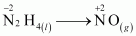Balance atom N:

N2H4(l)→2NO(g)

Add 8 electrons to balance oxidation no:

N2H4(l) → 2NO(g) + 8e

Add 8OH to balance the charge:

N2H4(l) + 8OH(aq) → 2NO(g) + 8e

Add 6 H2O to balance O atoms:

N2H4(l) + 8OH(aq) → 2NO(g) + 6H2O(l) + 8e  ——— (1)

The reduction half reaction:

ClO3(aq)  →  Cl(aq)

Add 6 electrons to balance oxidation no.

ClO3(aq)  +  6e→ Cl(aq)

Add 6OH– ions to balance the charge:

ClO3(aq) + 6e → Cl(aq) + 6OH(aq)

Add 3 H2O to balance O atoms:

ClO3(aq) + 3H2O(l) + 6e→ Cl(aq) + 6OH(aq) ——— (2)

Now, multiply the equation (1) by 3 and equation (2) by 4. Then, after adding them, we get the balanced redox reaction as given below:

3N2H4(l) + 4ClO3(aq) → 6NO(g)+4Cl(aq) + 6H2O(l)

Oxidation number method :

Reduction in the oxidation no. of N = 2 × 4 = 8

Increment in the oxidation no. of Cl = 1 × 6 = 6

Multiply N2H4 by 3 and ClO3 by 4 to balance the reduction and increment of the oxidation no. :

3N2H4(l) + 4ClO3(aq) → NO(g) + Cl(aq)

Balance Cl and n atoms :

3N2H4(l) + 4ClO3(aq)→ 6NO(g) + 4Cl(aq)

Add 6 H2O to balance O atoms :

3N2H4(l) + 4ClO3(aq) → 6NO(g) + 4Cl(aq) + 6H2O(l)

This is the required reaction equation.

(c)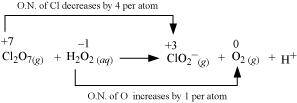The oxidation number of Cl decreases from + 7 in Cl2O7 to + 3 in ClO2 and the oxidation number of O increases from – 1 in H2O2 to zero in O2 .Hence, in this reaction, Cl2O7 is the oxidizing agent and H2O2 is the reducing agent.
Ion–electron method:
The oxidation half reaction:
H2O2(aq) → O2(g)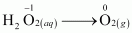Add 2 electrons to balance oxidation no:
H2O2(aq)→ O2(g) + 2e
Add 2OH to balance the charge:
H2O2(aq) + 2OH(aq) → O2(g)+2e
Add 2 H2O to balance O atoms:
H2O2(aq) + 2OH (aq)→ O2(g)+ 2H2O(l)+2e ——– (1)
The reduction half reaction: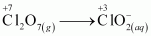Cl2O7(g)→ ClO2(aq)
Balance Cl atoms:
Cl2O7(g) → 2ClO2(aq)
Add 8 electrons to balance oxidation no.
Cl2O7(g) + 8e → 2ClO2(aq)
Add 6OH ions to balance the charge:
Cl2O7(g) + 8e→ 2ClO2(aq) + 6OH(aq)
Add 3 H2O to balance O atoms:
Cl2O7(g)+3H2O(l) + 8e→ 2ClO2(aq)+ 6OH(aq)
Now, multiply the equation (1) by 4. Then, adding equation (1) and (2), we get the balanced redox reaction as given below:
Cl2O7(g) + 4H2O2(aq) + 2OH(aq) → 2ClO2(aq) + 4O2(g) + 5H2O(l)
Oxidation number method :
Reduction in the oxidation no. of Cl2O7 = 4× 2 = 8
Increment in the oxidation no. of H2O= 2× 1 = 2
Multiply H2O2 by 4 and O2 by 4 to balance the reduction and increment of the oxidation no. :
3N2H4(l) + 4ClO3 (aq) → NO(g) + Cl(aq)
Balance Cl and n atoms:
Cl2O7(g) + 4H2O2(aq) → 2ClO – 2(aq)+ 4O2(g)
Add 3 H2O to balance O atoms:
Cl2O7(g)+4H2O2(aq) → 2ClO2(aq) + 4O2(g) + 3H2O(l)
Add 2OH and 2H2O to balance H atoms:
Cl2O7(g)+ 4H2O2(aq) 2OH(aq)→ 2ClO2(aq) + 4O2(g)+ 5H2O(l)
This is the required reaction equation.

20. What sorts of informations can you draw from the following reaction ?
(CN)2(g) + 2OH− (aq) ⟶ CN(g) + CNO(aq) + H2O(l)

The oxidation numbers of carbon in (CN)2,CN and CNO are +3, +2 and +4 respectively. These are obtained as shown below:
Let the oxidation number of C be x.
(CN)2

2(x – 3) = 0
∴x = 3
CN− x – 3 = –1
∴x = 2
CNO–x – 3 – 2 = –1
∴x = 4
The oxidation number of carbon in the various species is: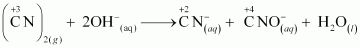It can be easily observed that the same compound is being reduced and oxidised simultaneously in the given equation. Reactions in which the same compound is reduced and oxidised is known as disproportionation reactions. Thus, it can be said that the alkaline decomposition of cyanogen is an example of disproportionation reaction.

21. The Mn3+ ion is unstable in solution and undergoes disproportionation to give  Mn2+ , MnO2 and Hion. Write a balanced ionic equation for the reaction.

The given reaction can be represented as:
Mn3+(αq)  ⟶ Mn2+(aq) + MnO2(s) + H+(aq)
The oxidation half equation is:
Mn3+(aq) ⟶ +4MnO2(s)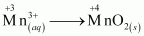The oxidation number is balanced by adding one electron as:
Mn3+(aq) ⟶ MnO2(s) +e
The charge is balanced by adding 4H+ ions as:
Mn3+(α) ⟶ MnO2(s) + 4H+(aq) + e
The O atoms and H+ ions are balanced by adding 2H2O molecules as:
The reduction half equation is:
Mn3+(aq) ⟶ Mn2+(aq)
The oxidation number is balanced by adding one electron as:
The balanced chemical equation can be obtained by adding equation (i) and (ii) as:

22. Consider the elements:
Cs, Ne, I and F
(a) Identify the element that exhibits only negative oxidation state.
(b) Identify the element that exhibits only positive oxidation state.
(c) Identify the element that exhibits both positive and negative oxidation states.
(d) Identify the element which exhibits neither the negative nor does the positive oxidation state.

(a) F exhibits only negative oxidation state of –1.
(b) Cs exhibits positive oxidation state of +1.
(c) I exhibits both positive and negative oxidation states. It exhibits oxidation states of – 1, + 1, + 3, + 5, and + 7.
(d) The oxidation state of Ne is zero. It exhibits neither negative nor positive oxidation states.

23. Chlorine is used to purify drinking water. Excess of chlorine is harmful. The excess of chlorine is removed by treating with sulphur dioxide. Present a balanced equation for this redox change taking place in water.

The redox reaction is as given below:

Cl2(s)+ SO2(aq) +H2O(l) → Cl(aq) + SO42−(aq)

The oxidation half reaction:
SO2(aq) → SO42−(aq)
Add 2 electrons to balance the oxidation no. :
SO2(aq)→ SO42−(aq)+ 2e

Add 4H+ ions to balance the charge:
SO2(aq)→ SO42−(aq)+ 4H+ (aq)+2e
Add 2 H2O to balance O atoms and H+ ions:
SO2(aq) + 2H2O → SO42−(aq) + 4H+(aq)+2e ——— (1)

The reduction half reaction:
Cl2(s)→ Cl(aq)

Balance Cl atoms:
Cl2(s)→ 2Cl(aq)
Add 2 electrons to balance the oxidation no. :
Cl2(s)+ 2e→ 2Cl(aq) ——— (2)
Add equation (1) and (2) to get the balanced chemical equation:
Cl2(s)+ SO2(aq)+ 2H2O(l) → 2Cl(aq)+ SO42−(aq) + 4H+(aq)

24. Refer to the periodic table given in your book and now answer the following questions:
(a) Select the possible non metals that can show disproportionation reaction.
(b) Select three metals that can show disproportionation reaction.

In disproportionation reactions, one of the reacting substances always contains an element that can exist in at least three oxidation states,
(a) P, Cl, and S can show disproportionation reactions as these elements can exist in three or more oxidation states,
(b) Mn, Cu, and Ga can show disproportionation reactions as these elements can exist in three or more oxidation states.

25. In Ostwald’s process for the manufacture of nitric acid, the first step involves the oxidation of ammonia gas by oxygen gas to give nitric oxide gas and steam. What is the maximum weight of nitric oxide that can be obtained starting only with 10.00 g. of ammonia and 20.00 g of oxygen?

The balanced chemical equation for the given reaction is given as:

Thus, 68 g of NH3 reacts with 160 g of O2

Therefore, 10g of NH3 reacts with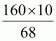g of O2, or 23.53 g of O2.
But the available amount of Ois 20 g.
Therefore, Ois the limiting reagent (we have considered the amount of O2 to calculate the weight of nitric oxide obtained in the reaction).
Now, 160 g of O2 gives 120g of NO.
Therefore, 20 g of O2 gives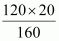g of N, or 15 g of NO.
Hence, a maximum of 15 g of nitric oxide can be obtained.

26. Using the standard electrode potentials given in the Table 8.1, predict if the reaction between the following is feasible:
(a) Fe3+(aq) and I(aq)
(b) Ag+(aq) and Cu(s)
(c) Fe3+(aq) and Cu(s)
(d) Ag(s) and Fe3+(aq)
(e) Br2(aq) and Fe2+(aq)

(i) Fe3+(aq) and I(aq)
2Fe3+(aq) + 2I(aq) → 2Fe2+(aq)+I2(s)

Oxidation half reaction: 2I(aq) → I2(s)+2e ;E∘=−0.54V
Reduction half reaction: [Fe3+(aq) + e → Fe2+(aq)]×2 ; E∘=+0.77V
——- ———– ——– ———- —— ———- —— ——— ———– —————-
2Fe3+(aq) + 2I→ 2Fe2+(aq)+ I2(s) ; E∘=+0.23V
Efor the overall reaction is positive. Therefore, the reaction between Fe3+(aq) and I(aq) is feasible.

(ii) Ag+(aq) and Cu(s)
2Ag+(aq)+Cu(s) → 2Ag(s) + Cu2+(aq)

Oxidation half reaction: Cu(s) → Cu2+(aq)+ 2e;  E=−0.34V
Reduction half reaction: [Ag+(aq)+e → Ag(s)]×2; E= +0.80V
——— ———– ———— ————– ———– ———- ————– —-

2Ag + (aq)+ Cu(s)→2Ag(s)+Cu2+; E∘=+0.46V
E for the overall reaction is positive. Therefore, the reaction between Ag+(aq) and Cu(s) is feasible

(iii) Fe3+(aq) and Cu(s)
2Fe3+(aq)+ Cu(s)→2Fe2+(s) + Cu2+(aq)

Oxidation half reaction: Cu(s)→ Cu2+(aq)+ 2e;   E∘=−0.34V
Reduction half reaction: [Fe3+(aq) + e→ Fe2+(s)] ×2;   E∘=+0.77V
—- ——— ———- ———– ———- ——— ——— ——- ————
2Fe3+(aq)+ Cu(s)→ 2Fe2+(s) + Cu2+(aq);             E=+0.43V
E for the overall reaction is positive. Therefore, the reaction between Fe3+(aq)and Cu(s) is feasible.

(iv) Ag(s) and Fe3+(aq)
Ag(s)+2Fe3+(aq)→ Ag+(aq)+ Fe2+(aq)

Oxidation half reaction: Ag(s)→ Ag+(aq)+e;   E∘=−0.80V
Reduction half reaction: Fe3+(aq) + e→Fe2+(aq); E∘=+0.77V
——- ———– ——– ——- ———– ——– ——- ——- ——- ———
Ag(s)+Fe3+(aq)→ Ag+(aq) + Fe2+(aq);             E=−0.03V

E for the overall reaction is positive. Therefore, the reaction between Ag(s) and Fe3+(aq) is feasible.

(v) Br2(aq) and Fe2+(aq)

Br2(s)+ 2Fe2+(aq) → 2Br(aq) + 2Fe3+(aq)
Oxidation half reaction: [Fe2+(aq) → Fe3+(aq) +e] ×2;               E= −0.77V
Reduction half reaction: Br2(aq)+2e→2Br(aq);                     E= +1.09V
———————————————————————————————————
Br2(s)+ 2Fe2+(aq) → 2Br(aq) + 2Fe3+(aq);                  E=−0.32V
E∘ for the overall reaction is positive. Therefore, the reaction between Br2(aq) and Fe2+(aq) is feasible.

27. Predict the products of electrolysis in each of the following:
(i) An aqueous solution of AgNO3 with silver electrodes
(ii) An aqueous solution AgNO3 with platinum electrodes
(iii) A dilute solution of H2SO4 with platinum electrodes
(iv) An aqueous solution of CuCl2 with platinum electrodes.

(i) AgNO3 ionizes in aqueous solution to form Ag+ and NO3 ions.
On electrolysis, either Ag+ ion or H2O molecule can be decreased at cathode. But the reduction potential of Ag+ ions is higher than that of H2O.
Ag+(aq) + e→Ag(s);  E=+0.80V
2H2O(l)+2e→ H2(g)+2OH(aq); E∘=−0.83V
Therefore, Ag+ ions are decreased at cathode. Same way, Ag metal or H2O molecules can be oxidized at anode. But the oxidation potential of Ag is greater than that of H2O molecules.
Ag(s)→ Ag+(aq) + e; E=−0.80V
2H2O(l) → O2(g)+ 4H + (aq) + 4e; E∘=−1.23V
Hence, Ag metal gets oxidized at anode.

(ii) Pt cannot be oxidized very easily. Therefore, at anode, oxidation of water occurs to liberate O2. At the cathode, Ag+ ions are decreased and get deposited.

(iii) H2SO4 ionizes in aqueous solutions to give H+ and SO42− ions.
H2SO4 (aq)  → 2H+(aq) + SO42−(aq)
On electrolysis, either of H2O molecules or H+ ions can get decreased at cathode. But the decreased potential of H+ ions is higher than that of H2O molecules.
2H+(aq) + 2e→ H2(g); E=0.0V
2H2O(aq) + 2e→ H2(g)+2OH(aq); E=−0.83V
Therefore, at cathode, H+ ions are decreased to free H2 gas.
On the other hand, at anode, either of H2O molecules or SO42− ions can be oxidized. But the oxidation of SO42− involves breaking of more bonds than that of H2O molecules. Therefore, SO42− ions have lower oxidation potential than H2O. Hence, H2O is oxidized at anode to free O2 molecules.

(iv)  In aqueous solutions, CuCl2 ionizes to give Cu2+ and Cl ions as:
CuCl2(aq)→ Cu2+(aq) + 2Cl(aq)
CuCl2(aq)→Cu2+(aq)+2Cl(aq)
On electrolysis, either of Cu2+ ions or H2O molecules can get decreased at cathode. But the decreased potential ofCu2+ is more than that of H2O molecules.
Cu 2+ (aq)+2e → Cu(aq); E∘=+0.34V
H2O(l) + 2e→H2(g)+ 2OH; E=−0.83V
Therefore, Cu2+ ions are decreased at cathode and get deposited. In the same way, at anode, either of Cl orH2O is oxidized. The oxidation potential of H2O is higher than that of Cl.
2Cl(aq)→ Cl2(g) + 2e; E=+0.34V
2H2O(l) → O2(g) + 4H+(aq)+ 4e; E=−1.23V
But oxidation of H2O molecules occurs at a lower electrode potential compared to that of Cl− ions because of over-voltage (extra voltage required to liberate gas). As a result, Cl− ions are oxidized at the anode to liberate Cl2 gas.

28. Arrange the given metals in the order in which they displace each other from the solution of their salts.
(i) Al
(ii) Fe
(iii) Cu
(iv) Zn
(v) Mg

A metal with stronger reducing power displaces another metal with weaker reducing power from its solution of salt.
The order of the increasing reducing power of the given metals is as given below:
Cu < Fe < Zn < Al < Mg
Therefore, Mg can displace Al from its salt solution, but Al cannot displace Mg. Thus, the order in which the given metals displace each other from the solution of their salts is as given below: Mg >Al>Zn> Fe >Cu

29. The standard electrode potentials are given of the following elements:
K+/K  = –2.93V
Ag+/Ag  = 0.80V
Hg2+/Hg  = 0.79V
Mg2+/Mg  = –2.37V
Cr3+/Cr  = –0.74V
Arrange these metals in their increasing order of reducing power.

The reducing agent is stronger as the electrode potential decreases. Hence, the increasing order of the reducing power of the given metals is as given below:
Ag < Hg < Cr < Mg < K

30. Depict the galvanic cell in which the reaction is:
Zn(s) + 2Ag+(aq)→ Zn2+(aq) + 2Ag(s)
Show the following:
(i) Which of the electrode is negatively charged?
(ii) Name the carriers of the current in the cell.
(iii) Write the individual reaction at each electrode.

The galvanic cell corresponding to the given redox reaction can be shown as:
Zn|Zn2+(aq)|| Ag+(aq)|Ag

(i) Zn electrode is negatively charged because at this electrode, Zn oxidizes to Zn2+ and the leaving electrons accumulate on this electrode.

(ii) The carriers of current are ions in the cell.

(iii) Reaction at Zn electrode is shown as:
Zn(s)→ Zn2+(aq) + 2e
Reaction at Ag electrode is shown as:
Ag+(aq) + e→ Ag(s)

## Conclusions for NCERT SOLUTIONS FOR CLASS 11 CHEMISTRY CHAPTER 8-REDOX REACTIONS

The NCERT Solutions for Class 11 Chemistry Chapter 8 – Redox Reactions offered by Swastik Classes are an invaluable resource for students seeking a comprehensive understanding of this important topic. Our solutions have been meticulously crafted to provide clarity and support in mastering the concepts and principles of redox reactions.

By engaging with our solutions, students can develop a strong foundation in oxidation-reduction reactions, oxidation numbers, and balancing redox equations using the ion-electron method. The step-by-step explanations, examples, and practice questions enable students to enhance their problem-solving skills and gain confidence in tackling a variety of questions.

Swastik Classes aims to make the learning experience enjoyable and accessible. Our NCERT Solutions simplify complex concepts, foster critical thinking, and stimulate curiosity in the captivating field of chemistry. We strive to instill a lifelong love for the subject and empower students to excel academically.

With the assistance of our experienced educators and subject matter experts, students can effectively grasp the theoretical aspects of redox reactions while also developing their analytical and reasoning abilities. The solutions are designed to cater to the needs of students and educators alike, offering a reliable and comprehensive guide for academic success.

Swastik Classes is committed to providing top-quality educational resources, and our NCERT Solutions for Class 11 Chemistry Chapter 8 – Redox Reactions exemplify our dedication to excellence. Let Swastik Classes be your trusted companion on your educational journey, helping you unlock the mysteries of redox reactions and achieve academic excellence.

1. What is the importance of redox reactions in everyday life? Redox reactions play a vital role in various aspects of everyday life. They are involved in processes such as combustion, respiration, photosynthesis, corrosion of metals, and even in the functioning of batteries and fuel cells. Redox reactions are crucial for energy production, metabolism, and the maintenance of environmental balance.
2. How can I determine the oxidation number of an element in a compound? The oxidation number of an element in a compound can be determined by following a set of rules. For example, the oxidation number of an uncombined element is always zero, while in a compound, the sum of oxidation numbers is equal to the overall charge. Additionally, certain elements have fixed oxidation numbers (e.g., oxygen is usually -2), while others have variable oxidation numbers based on the surrounding elements.
3. What is the ion-electron method for balancing redox reactions? The ion-electron method, also known as the half-reaction method, is used to balance redox equations. In this method, the oxidation and reduction half-reactions are balanced separately by considering the transfer of electrons. The number of electrons transferred in each half-reaction is adjusted so that the total number of electrons gained equals the total number of electrons lost. Finally, the balanced half-reactions are combined to obtain the balanced redox equation.
4. How can I identify whether a reaction is a redox reaction? A redox reaction involves the transfer of electrons between species. To identify whether a reaction is a redox reaction, you can analyze the change in oxidation numbers of the elements involved. If the oxidation number of an element increases, it undergoes oxidation, and if the oxidation number decreases, it undergoes reduction. If there is a change in oxidation numbers, the reaction is a redox reaction.
5. Are there any real-life applications of balancing redox reactions? Yes, balancing redox reactions has several practical applications. It is essential in industries such as pharmaceuticals, chemical manufacturing, and environmental analysis. Balancing redox reactions allows scientists to determine the stoichiometry of reactants and products accurately, ensuring efficient and controlled chemical processes. Additionally, it is crucial in designing and optimizing chemical reactions for various applications, such as drug synthesis and wastewater treatment.